# Important Questions for Class 12 - Physics - Chapter 2 - Electrostatic Potential and Capacitance

If an external force does work to move a body from one point to another while opposing a force, such as a gravitational force or spring force, the work is converted into the potential energy of the body. When the external force is no longer applied, the body will start moving, gaining kinetic energy while losing an equal amount of potential energy. The sum of kinetic and potential energy is preserved in this process. These types of forces are called conservative forces, and spring force and gravitational force are examples of such forces.

The Coulomb force between two stationary charges is conservative. This is unsurprising, as both the Coulomb force and gravitational force exhibit an inverse-square dependence on distance and differ mainly in their proportionality constants (charge in Coulomb’s law versus mass in the gravitational law). Therefore, similar to how we can define the potential energy of a mass in a gravitational field, we can also define the electrostatic potential energy of a charge in an electrostatic field.

To define the potential energy of a test charge q, we consider the work done on the charge q. The work is proportional to q; the value of force at any point is equal to qE, where E is the electric field at that point due to the given charge configuration. Therefore, it is convenient to divide the work by the magnitude of the charge q, resulting in a quantity that is independent of q. In other words, the work done per unit charge is a characteristic of the electric field associated with the charge configuration.

We are now considering the potential energy of a charge or charges in an external field rather than the field produced by the charge itself. The external field, denoted as E, is produced by sources outside of the charge being considered and is not produced by the charge itself. In many cases, the sources of the external field are unknown or unspecified. However, the electric field E or the electrostatic potential V caused by these external sources is specified. It is assumed that the charge q does not significantly alter the external sources that produce the field. This assumption is valid if the charge q is very small or if the external sources are fixed in place by other forces. Even if q is not infinitesimally small, it may still be assumed to have no significant impact on the external sources if there are strong sources far away that produce a finite field E in the region of interest. This is because the influence of q on external sources may be negligible in comparison to strong sources.

It is important to reiterate that our focus is on determining the potential energy of a specific charge q (or system of charges) in an external field rather than the potential energy of the sources that produce the external field. The external electric field E and the associated external potential V can vary from one point to another. The potential V at point P is defined as the work required to bring a unit positive charge from infinity to point P. (We maintain the convention of taking the potential at infinity to be zero.) Therefore, the work required to bring a charge q from infinity to the point P in an external field is qV, and this work is stored as the potential energy of the charge q.

Important Questions for Class 12 Physics Chapter 2 – Electrostatic Potential and Capacitance are provided here. Students must go through these questions and solve them to prepare for their Physics papers. They can also refer to these questions for quick revision. These questions are more likely to be asked in the exam; hence, students must practise them thoroughly.

## Very Short Answer Type Questions

1. Consider two conducting spheres of radii R1 and R2 with R1 > R2. If the two are at the same potential, the larger sphere has more charge than the smaller sphere. State whether the charge density of the smaller sphere is more or less than that of the larger one.

Solution:

In this case, V1 = V2

$$\begin{array}{l}\frac{q_1}{4 \pi \varepsilon_0 R_1 } = \frac{q_2}{4 \pi \varepsilon_0 R_2 }\end{array}$$
$$\begin{array}{l}\textup{Therefore, } \frac{q_1}{q_2} = \frac{R_1}{R_2} \,\,\,…. \textup{(i)}\end{array}$$

It is given that R1 > R2

$$\begin{array}{l}\textup{Therefore, } q_1 > q_2\end{array}$$

Therefore, the bigger sphere has more electric charge than the smaller sphere. Then the charge densities

$$\begin{array}{l}\sigma_1 = \frac{q_1}{4 \pi R^2_1 }, \,\,\, \sigma_2 = \frac{q_2}{ 4 \pi R^2_2 }\end{array}$$
$$\begin{array}{l}\textup{Therefore, } \frac{\sigma_2 }{\sigma_1} = \frac{q_2R^2_1}{q_1R^2_2} \end{array}$$
$$\begin{array}{l}\frac{ \sigma_2 }{ \sigma_1 } = \frac{ R_2 }{ R_1 } \frac{R_1^2}{ R^2_2 } = \frac{R_1}{R_2} (\textup{applying (i)})\end{array}$$
$$\begin{array}{l}\textup{Since } R_1 > R_2, \,\,\, \therefore \sigma_1 > \sigma_2 \end{array}$$

The charge density of a smaller sphere is greater than that of the larger sphere.

2) Do free electrons travel to regions of higher potential or lower potential?

Solution: The free electrons experience an electrostatic force in the opposite direction of the electric field due to their negative charge. Since the electric field always moves from higher potential to lower potential, the electrostatic force and the direction of travel of the electrons is from the region of lower potential to the region of higher potential.

3) Can there be a potential difference between two adjacent conductors carrying the same charge?

Solution:

From the mathematical relation Q = CV

$$\begin{array}{l}V_1 = \frac{Q_1}{C_1} \textup{ and } V_2 = \frac{Q_2}{C_2}\end{array}$$
$$\begin{array}{l}\frac{V_1}{V_2} = \frac{C_2}{C_1}\end{array}$$

So, the potential difference across a conductor depends not only on the charge present but also on the geometry of the conductor, which influences its capacitance. As a result, two adjacent conductors with the same charge but different dimensions may have different potential differences.

4) Can the potential function have a maximum or minimum in free space?

Solution:

The potential function does not have a maximum or minimum value in free space because there is no atmosphere present to cause electric discharge or potential leakage around the conductor.

5) A test charge q is made to move in an electric field of a point charge Q along the two different closed paths. The first path has sections along and perpendicular to the lines of an electric field. The second path is a rectangular loop of the same area as the first loop. How does the work done compare in the two cases?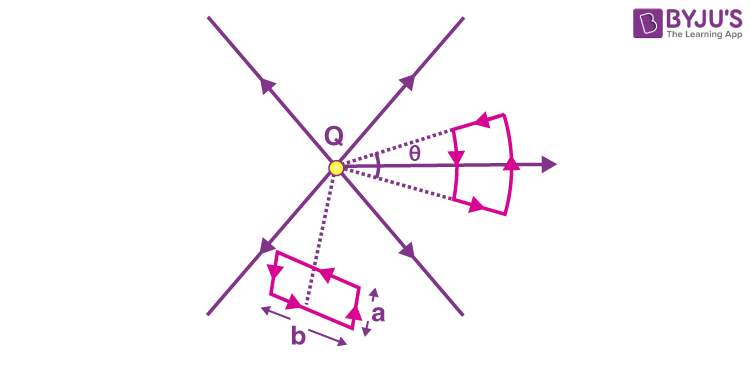Solution:

The work done by an electrostatic force is conservative, which means that the work done in a closed loop is always zero, regardless of the path taken within the loop.

1) Prove that a closed equipotential surface with no charge within itself must enclose an equipotential volume.

Solution:

Assuming a contradicting statement, the potential within a closed equipotential surface is not uniform. Let the potential just inside the surface be different to that on the surface having a potential gradient (dV/dr).
Therefore, an electric field is created, which is represented by the following:

E=−dV/dr

As a result, field lines either point inward or outward from the surface. These lines cannot originate from the surface itself, as it is equipotential. Field lines can only be formed when they have a charge at the other end within the surface. This conclusion contradicts the initial assumption. Therefore, it must be the case that the entire volume inside is equipotential.

2) A capacitor has some dielectric between its plates, and the capacitor is connected to a DC source. The battery is now disconnected, and then the dielectric is removed. State whether the capacitance, the energy stored in it, the electric field, the charge stored, and the voltage will increase, decrease or remain constant.

Solution:

When a capacitor is connected to a DC source, it becomes charged. When the battery is disconnected, no more charge can flow into the capacitor. Removing the dielectric material from the capacitor will cause its capacitance to decrease.

$$\begin{array}{l}\textup{Energy stored will rise } \left ( u = \frac{q^2}{2C} \right )\end{array}$$
$$\begin{array}{l}\textup{Potential will also rise } \left ( V = \frac{q}{C} \right )\end{array}$$
$$\begin{array}{l}\textup{Electric field will rise } \left ( E = \frac{V}{D} \right )\end{array}$$

3) Prove that if an insulated, uncharged conductor is placed near a charged conductor and no other conductors are present, the uncharged body must be intermediate in potential between that of the charged body and that of infinity.

Solution:

Imagine that a charged body, designated as A, is placed near an insulated, uncharged conductor, designated as B. A has a positive charge. The setup is shown in the accompanying figure.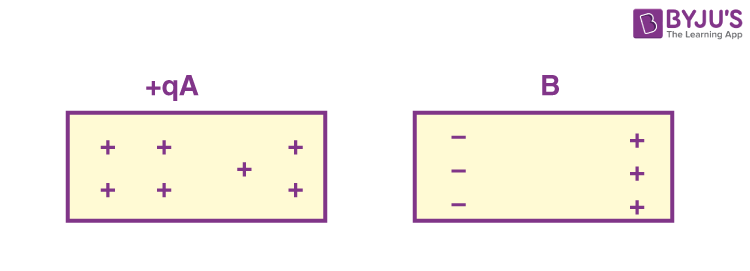$$\begin{array}{l}\textup{As } V = \frac{kq}{r} \textup{ k and q are constants, so }\end{array}$$
$$\begin{array}{l}V \alpha \frac{1}{r}\end{array}$$

The uncharged conductor is situated between the charged conductor and infinity, causing the potential to decrease from body A to infinity. As a result, the potential of the uncharged body varies between the potential of A and infinity.

4) Calculate the potential energy of a point charge -q placed along the axis due to a charge +Q uniformly distributed along a ring of radius R. Sketch P.E as a function of axial distance z from the centre of the ring. Looking at the graph, can you see what would happen if -q is displaced slightly from the centre of the ring (along the axis)?

Solution:

The potential energy (U) of a point charge q at a potential V is given by U=qV. In this case, a negatively charged particle is located on the axis of a ring with charge Q. The ring has a radius of a, and the electric potential at a distance x from the centre of the ring along the axis can be calculated.

The graph in Fig. shows how the potential energy changes as a function of axial distance z from the centre of the ring. If a charge of -q is slightly displaced from the centre of the ring along the axis and released, it will undergo oscillations. However, it is not possible to determine the nature of these oscillations simply by examining the graph.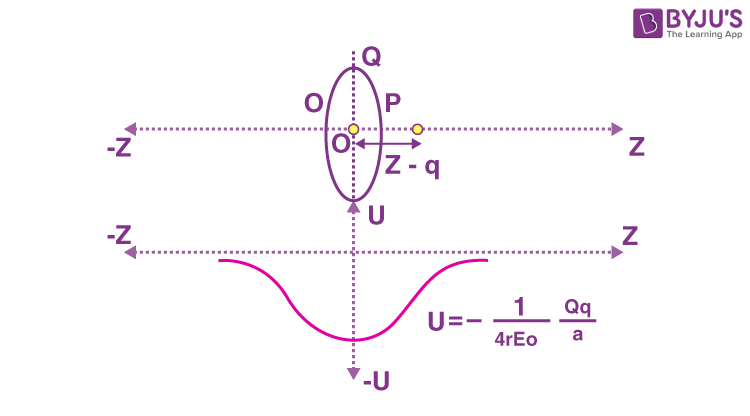5) Calculate potential on the axis of a ring due to charge Q uniformly distributed along the ring of radius R.

Solution: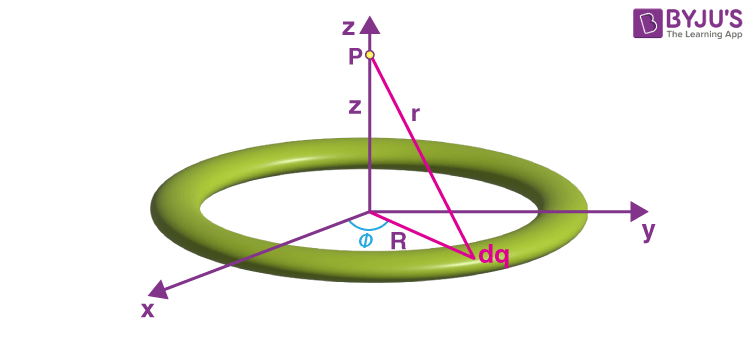Let’s assume a ring of radius R possessing charge +Q that is evenly distributed. A point P at length z passes through the centre O, and it is perpendicular to the ring’s plane.

Next, assume an element of the ring at point S (length di) possessing a charge dq and SP is equivalent to r.

Consequently, the potential energy due to element r and the potential energy due to element dl at p

$$\begin{array}{l}dV = \frac{-kdq}{r} \textup{ where k =} \frac{1}{4 \pi \varepsilon_0}\end{array}$$

Charge on the 2πR length of ring is equal to Q.

$$\begin{array}{l}\textup{Charge ion dl length} = \frac{Q}{2 \pi R }dl \end{array}$$

Therefore, at P, the potential due to element di is

$$\begin{array}{l}dV = \frac{-kQdl}{2\pi R}\end{array}$$

By integrating over the ring potential Vp at point P ,

$$\begin{array}{l}\int_{0}^{V} dV_p = \int_{0}^{2\pi R} \frac{kQdl}{2 \pi Rr} \end{array}$$
$$\begin{array}{l}r = \sqrt{R^2 + z^2}\end{array}$$
$$\begin{array}{l}V_p = \frac{kQ2 \pi R}{2\pi R \sqrt{R^2 + z^2}}\end{array}$$
$$\begin{array}{l}= \frac{Q}{ 4 \pi \varepsilon_0 \sqrt{R^2 + z^2} }\end{array}$$
$$\begin{array}{l}\textup{Charge on dl length} = \frac{Q}{2 \pi R } dl\end{array}$$
$$\begin{array}{l}\textup{Charge on dl length} = \frac{Q}{2 \pi R } dl\end{array}$$

Therefore, the potential caused by element di at point P is

$$\begin{array}{l}dV = \frac{-kQdl}{2 \pi R}\end{array}$$

By integrating over the ring the potential Vp at point P, we get

$$\begin{array}{l}\int_{0}^{V} dV_p = \int_{0}^{2mR} \frac{kQa^1}{ 2 \pi Rr } \,\,\, \textup{where r = } \sqrt{R^2 + z^2}\end{array}$$
$$\begin{array}{l}V_p = \frac{ kQ2 \pi R }{ 2 \pi R \sqrt{ R^2 +z^2 } }\end{array}$$
$$\begin{array}{l}= \frac{ Q }{ 4 \pi \varepsilon_0 \sqrt{ R^2 + z^2} }\end{array}$$

1) Find the equation of the equipotentials for an infinite cylinder of radius r0, carrying charge of linear density λ.

Solution: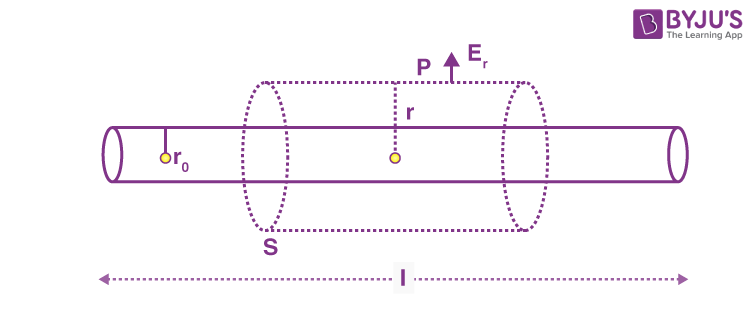Take the case of a cylindrical Gaussian dotted surface (S) at a length r from the cylinder’s centre of radius r0 of infinite length. The electric field lines are perpendicular and radial to the surface.

Consider electric field intensity on the given Gaussian surface at point P is E, and net charge q on the cylinder be q=λl

Thus, by applying Gauss’s law,

$$\begin{array}{l}\oint_{s} E_r ds = \frac{ \lambda l }{ \varepsilon_0 }\end{array}$$
$$\begin{array}{l}\Rightarrow [E_r cos \theta]^{2 \pi i} _0 = \frac{ \lambda l }{ \varepsilon_0 }\end{array}$$

In this scenario, ‘ε0’ is the free space’s permittivity, ‘l’ is the length of the cylinder, ‘q’ is the charge bounded within the surface, and ‘S’ is the area vector perpendicular to the surface. ‘2π rl’ is the area of the curved cylindrical surface.

$$\begin{array}{l}E_r \, 2 \pi rl\,\cos90 ^ \circ = \frac{ \lambda }{ \varepsilon_0 } \, [ \angle \theta ] \textup{ is between } E_r \textup{ curved surface of the dotted cylinder is }90^\circ J. \end{array}$$
$$\begin{array}{l}E_r = \frac{ \lambda }{ 2 \pi r \varepsilon_0 }\end{array}$$

When r0 is the infinite cylinder’s radius,

$$\begin{array}{l}V (r) – V (r_0) = – \int_{r_{0}} ^{r} E.dl \end{array}$$
$$\begin{array}{l}= – \int _{r_0} ^{r} \frac{ \lambda }{ 2 \pi \varepsilon_0 r } dr\end{array}$$
$$\begin{array}{l}V(r) – V(r_0) = – \frac{ \lambda }{ 2 \pi \varepsilon_0} ( In(r) – In (r_0) )\end{array}$$
$$\begin{array}{l}V(r) – V(r_0) = – \frac{ \lambda }{ 2 \pi \varepsilon_0 } In(r/r_0)\end{array}$$

In the case of the given V,

$$\begin{array}{l}In (r/r_0) = – \frac{ 2 \pi \varepsilon_0 }{ \lambda } [V(r) – V(r_0)]\end{array}$$
$$\begin{array}{l}r = r_0 \, e^{-2} \pi \varepsilon_0 [V(r) – V_{(r_0)}]/ \lambda\end{array}$$

Therefore, equipotential surfaces are the coaxial curved surfaces of cylinders with the given cylinder of radius r.

2) Two point charges of magnitude +q and -q are placed at (-d/2, 0,0) and (d/2, 0,0), respectively. Find the equation of the equipotential surface where the potential is zero.

Solution: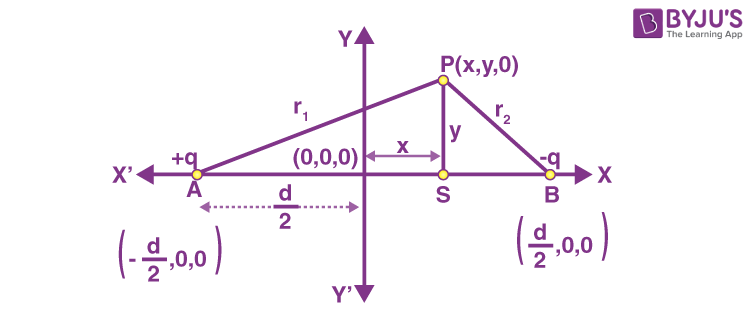The potential caused by charges -q and +q are zero in between the line connecting the two charges -q and +q. Consider zero potential is at point S. Then the equipotential surface will go through S and normal to the line connecting the two charges (AB).

$$\begin{array}{l}\textup{ Therefore, }r_1^2 = AS^2 + SP^2 = \left ( x + \frac{d}{2} \right )^2 +y^2\end{array}$$
$$\begin{array}{l}r_1 = \sqrt{ \left ( x + \frac{d}{2} \right ) }^2 + y^2\end{array}$$

Likewise,

$$\begin{array}{l}r_2 = \sqrt{ \left ( x – \frac{d}{2} \right )^2 +y^2 }\end{array}$$

Therefore, the total potential at point P = 0

$$\begin{array}{l}\frac{kq}{ r_1 } + \frac{k(-q)}{ r_2 } = 0 \textup{ where, } k= \frac{1}{ 4 \pi \varepsilon_0 }\end{array}$$
$$\begin{array}{l}\Rightarrow \left [ \frac{1}{r_1} – \frac{1}{r_2} \right ] = 0 \, [\because kq = 0]\end{array}$$
$$\begin{array}{l}\therefore \frac{1}{r_1} – \frac{1}{r_2} = 0\end{array}$$
$$\begin{array}{l}\frac{1}{r_1} = \frac{1}{r_2} \Rightarrow r_1 = r_2\end{array}$$
$$\begin{array}{l}\left ( x + \frac{d}{2} \right )^2 + y^2 = \left ( x- \frac{d}{2} \right )^2 + y^2 \end{array}$$
$$\begin{array}{l}\Rightarrow x^2 + \frac{d^2}{4} + dx = x^2 + \frac{d^2}{4} – dx\end{array}$$
$$\begin{array}{l}2dx = 0\end{array}$$
$$\begin{array}{l}2d = 0\end{array}$$
$$\begin{array}{l}\textup{Therefore, } x = 0 \end{array}$$

Thus, the equipotential surface will be normal to the x-axis going through x = 0 (origin in y-z plane).

3) A parallel plate capacitor is filled by a dielectric whose relative permittivity varies with the applied voltage (U) as ε = αU where α = 2V-1. A similar capacitor with no dielectric is charged U0 = 78 V. It is then connected to the unchanged capacitor with the dielectric. Find the final voltage on the capacitors.

Solution:

After connecting the two capacitors, let’s consider the final voltage to be ‘V’.
When the capacitance of the capacitor with dielectric is C, the charge on the capacitor is q1 (equal to CV). Let’s also assume that the other capacitor with dielectric possesses εC capacitance.

So, the charge on it is

$$\begin{array}{l}q_2 = \varepsilon CV\end{array}$$

SInce ε = αV, q2 = ε = αV

The initial charge on the given capacitor (without the dielectric) that was electrically charged is

$$\begin{array}{l}q_0 = CV_0\end{array}$$

From the law of conservation charges,

$$\begin{array}{l}q_0 = q_1 + q_2\end{array}$$
$$\begin{array}{l}CV_0 = CV + \alpha CV^2 \end{array}$$
$$\begin{array}{l}V^2 + V – V_0 = 0\end{array}$$
$$\begin{array}{l}V = \frac{ -1 \pm \sqrt{1 + 4 \alpha V_0} }{2 \alpha }\end{array}$$
$$\begin{array}{l}\textup{Applying } \alpha = 2V^{-1} \textup{ or } V_0 = 78 V,\textup{ we get}\end{array}$$
$$\begin{array}{l}V = \frac{ -1 \pm \sqrt{ 1 + (4 \times 2 \times 78 ) } }{2 \times 2}\end{array}$$
$$\begin{array}{l}= \frac{ -1 \pm \sqrt{ 625 } }{4}\end{array}$$

Since V is positive,

$$\begin{array}{l}V = \frac{ \sqrt{625} -1 }{4} = \frac{24}{4} = 6V\end{array}$$

4) A capacitor is made of two circular plates of radius R each, separated by a distance d << R. The capacitor is connected to a constant voltage. A thin conducting disc of radius r << R and thickness t << r is placed at the centre of the bottom plate. Find the minimum voltage required to lift the disc if the mass of the disc is m.

Solution:

$$\begin{array}{l}\textup{Electric field on the disc E =} V/d\end{array}$$

Thus, the charge ‘q’ transferred to the disc is

$$\begin{array}{l}q = CV = \left ( \frac{ \varepsilon_0A }{d} \right ) V = \varepsilon_0 \frac{V}{d} \pi r^2 \end{array}$$

Then, the force acting on the disc is

$$\begin{array}{l}F = qE = \left ( \frac{ \varepsilon_0 A }{d} \pi r^2 \right ) \frac{V}{d}\end{array}$$
$$\begin{array}{l}F = \varepsilon_0 \frac{V^2}{d^2} \pi r^2\end{array}$$

When the disc is lifted,

$$\begin{array}{l}F \geq mg\end{array}$$
$$\begin{array}{l}\varepsilon_0 \frac{V^2}{d^2} \pi r^2 = mg \textup{ (for minimum V) }\end{array}$$
$$\begin{array}{l}\Rightarrow V = \sqrt{ \frac{mgd^2}{ \pi \varepsilon_0 r^2 } } \end{array}$$

5) (a) In a quark model of elementary particles, a neutron is made of one up-quarks [charge (2/3)e] and two down-quarks [charges -(1/3)e]. Assume that they have a triangle configuration with a side length of the order of 10-15 m. Calculate the electrostatic potential energy of the neutron and compare it with its mass of 939 MeV.

(b) Repeat the above exercise for a proton which is made of two up and one down quarks.

Solution:

(a) Construct a rough figure.

The charge on an up-quark is

$$\begin{array}{l}q_{up} = \frac{2}{3e}\end{array}$$

The charge on a down-quark is

$$\begin{array}{l}q_{down} = – \frac{1}{3e}\end{array}$$

The length between each quark is

$$\begin{array}{l}d = 10^{-15}m\end{array}$$

The potential energy of the charge system is expressed by,

$$\begin{array}{l}U = \frac{1}{ 4 \pi \varepsilon_0 d } [q_{down}q_{down} + q_{down}q_{up} + q_{down}q_{up}]\end{array}$$
$$\begin{array}{l}e = 1.6 \times 10^{-19} C\end{array}$$
$$\begin{array}{l}U = \frac{ 1 }{ 4 \pi (8.854 \times 10^{-12})(10^{-15}) } (1.6 \times 10^{-19} )^2 [ \frac{1}{9} – \frac{4}{9} ]\end{array}$$
$$\begin{array}{l}U = – 76.81 \times 10^{-15} J = \frac{ -76.81 \times 10^{-15} }{1.6 \times 10^{19} eV}\end{array}$$
$$\begin{array}{l}(\because 1 eV = 1.6 \times 10^{-19}J)\end{array}$$

By comparing the resulted energy with the mass of the neutron (939 MeV), the potential energy is,

$$\begin{array}{l}\frac{ -0.48 }{ 939 } = 5.11 \times 10^{-4} neutron \,\, masses \end{array}$$

(a) Construct a rough figure.The charge on an up-quark is

$$\begin{array}{l}q_{up} = \frac{2}{3e}\end{array}$$

The charge on a down-quark is

$$\begin{array}{l}q_{down} = – \frac{1}{3e}\end{array}$$

The length between each quark is 10-15 m. The potential energy of the charge system is given by

$$\begin{array}{l}U = \frac{1}{ 4 \pi \varepsilon_0 r }[ q_{up}q_{up} + q_{down}q_{up} + q_{down}q_{up} ]\end{array}$$

Substitute the corresponding values, and then we get

$$\begin{array}{l}U = \frac{1}{ 4 \pi (8.854 \times 10^{-12})(10^{-15}) } [ \frac{2}{3}e(\frac{2}{2}e) + (-\frac{1}{3}e)(\frac{2}{3}e) + (-\frac{1}{3}e)(\frac{2}{3}e) ]\end{array}$$
$$\begin{array}{l}(e = 1.6 \times 10^{-19} C)\end{array}$$
$$\begin{array}{l}U = \frac{1}{ 4 \pi (8.854 \times 10^{-12}) (10^{-15}) } (1.6 \times 10^{-19})^2 [ \frac{4}{9} – \frac{4}{9} ]\end{array}$$
$$\begin{array}{l}= 0\, eV\end{array}$$

6) Two metal spheres, one of radius R and the other of radius 2R, both have the same surface charge density σ. They are brought in contact and separated. What will be the new surface charge densities on them?

Solution:

Before making contact, the charges of the two spheres are

$$\begin{array}{l}q_1 = \sigma 4 \pi R^2, \,\,\, q_2 = \sigma 4 \pi (2 R)^2 =4 q_1 \end{array}$$

If the two spheres are made to touch one another, their charges are shared till their potentials become the same value, V1 = V2

$$\begin{array}{l}\textup{Therefore, } \frac{q_1}{ 4 \pi \varepsilon_0 R } = \frac{ q_2 }{ 4 \pi \varepsilon_0 (2R) }\end{array}$$
$$\begin{array}{l}\Rightarrow q_2 = 2 q_1 \textup{ …..(i)} \end{array}$$

Since there is no loss of charge in the phenomenon

$$\begin{array}{l}q_1 + q_2 = q_1 + q_2 = q_1 +4q_1 = 5q_1 \end{array}$$
$$\begin{array}{l}= 5 ( \sigma 4 \pi R^2 )\end{array}$$
$$\begin{array}{l}q_1 + 2q_1 = 5 \sigma 4 \pi R^2 \,\,\, ( \textup{using (i)})\end{array}$$
$$\begin{array}{l}q_1 = \frac{ 5 }{3} \sigma 4 \pi R^2 ,\,\,\, q_2 = 2q_1 = \frac{10}{3} ( \sigma 4 \pi R^2 )\end{array}$$
$$\begin{array}{l}\therefore \sigma_1 = \frac{q_1}{ 4 \pi R^2 } = \frac{5}{3} \sigma \end{array}$$
$$\begin{array}{l}\therefore \sigma_2 = \frac{ q_2 }{ 4 \pi (2R)^2 } = \frac{5}{6} \sigma\end{array}$$

7. In the circuit shown in the diagram, initially, K1 is closed, and K2 is open. What are the charges on each capacitor? Then K1 was opened, and K2 was closed (order is important), what will be the charge on each capacitor now? [C = 1uF]?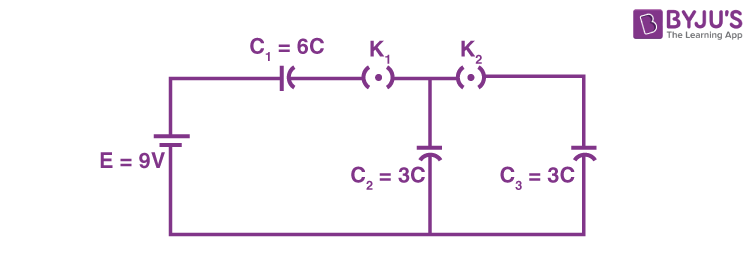Solution:

If K1 is closed and K2 is open, the capacitors C2 and C1 will charge, and potential arises across them V2 and V1, respectively, which will be equivalent to the potential of the 9V battery.

$$\begin{array}{l}\therefore V_1 + V_2 = 9 \textup{ ……(i)} \end{array}$$
$$\begin{array}{l}\therefore V = \frac{q}{C}\,\, \textup{ or } V \alpha \frac{1}{C}\end{array}$$
$$\begin{array}{l}\Rightarrow \frac{V_1}{V_2} = \frac{C_2}{C_1} \end{array}$$
$$\begin{array}{l}\frac{V_1}{V_2} = \frac{3C}{6C}\end{array}$$
$$\begin{array}{l}6V_1 = 3V_2\end{array}$$
$$\begin{array}{l}V_2 = 2 V_1 \textup{ …..(ii) }\end{array}$$

From the equations (i) and (ii)

$$\begin{array}{l}V_1 + 2V_1 = 9 \end{array}$$
$$\begin{array}{l}3 V_1 = 9\end{array}$$
$$\begin{array}{l}V_1 = 3 \textup{volt}\end{array}$$
$$\begin{array}{l}V_2 = 2 \times 3 \textup{volt} = 6 \textup{volt}\end{array}$$
$$\begin{array}{l}\Rightarrow q_1 = C_1 V_1 \,\,\,\, ( \textup{from the equation (ii)}) \end{array}$$
$$\begin{array}{l}6C \times 3 = 18 C\end{array}$$
$$\begin{array}{l}= 18 \times 1 \mu F =18 \mu c \,\,\,\, (C = 1 \mu F)\end{array}$$
$$\begin{array}{l}q_2 = C_2 V_2 = 3C \times 6\end{array}$$
$$\begin{array}{l}= 18 \mu C\end{array}$$

Therefore, the charges on each capacitor, q1 = q2 = 18μc

If k1 is open and k2 is closed off, then the charge q2 will be infused in C2 and C3. Let’s consider it as q2 and q3

$$\begin{array}{l}\Rightarrow q_2 = q_2 + q_3 \end{array}$$

Since C2 and C3 are connected parallelly, their potentials stay the same.

$$\begin{array}{l}\therefore q_2 = C_2V + C_3V \end{array}$$
$$\begin{array}{l}18 \mu C = 3 \times \mu F \times V +3 \times 1 \mu F \times V\end{array}$$
$$\begin{array}{l}18 = 6V\end{array}$$
$$\begin{array}{l}\Rightarrow V = 3 \textup{volt} \end{array}$$

Thus, the potential on C3 and C2 are 3 volts each.

$$\begin{array}{l}q_2 = C_2V = 3 \times 1 \mu F \times 3V \end{array}$$
$$\begin{array}{l}= 9 \mu C\end{array}$$
$$\begin{array}{l}q_3 = C_3V = 3 \times 1 \mu F \times 3V\end{array}$$
$$\begin{array}{l}= 9 \mu C\end{array}$$

8. Calculate potential on the axis or a disc or radius R due to a charge Q uniformly distributed on its surface.

Solution: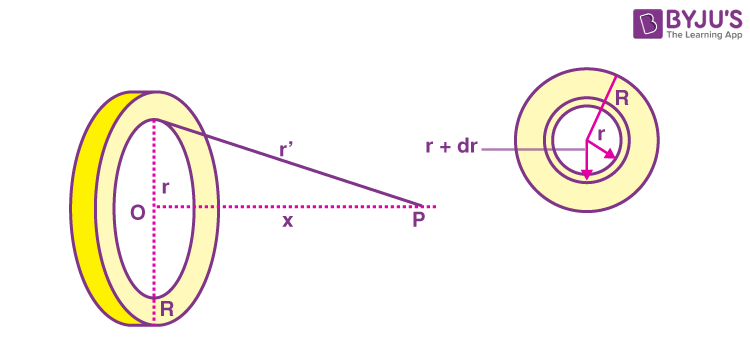Take a point P on the axis normal to the disc’s plane and length ‘x’ from the centre O as represented in the diagram. Then, take a ring of radius r with thickness dr on the disc with radius R, as represented in the above diagram. Also, consider the charge on the ring is dq. Then the potential dV due to ring at point P will be

$$\begin{array}{l}dV = \frac{kdq}{r} \,\,\,\,\,\, [\textup{since } r’= \sqrt{ r^2 + x^2 }]\end{array}$$

‘dq’ is the charge on the ring.

$$\begin{array}{l}dq = \sigma \times (\textup{ area of ring})\end{array}$$
$$\begin{array}{l}= \sigma \times [ \pi (r+dr)^2 – \pi r^2 ]\end{array}$$
$$\begin{array}{l}\Rightarrow dq = \sigma \times \pi [ r^2 + dr^2 + 2rdr – r^2 ] \end{array}$$

Since dr is very small, dr2 is practically negligible.

$$\begin{array}{l}dq = \sigma \pi (2rdr)\end{array}$$
$$\begin{array}{l}= 2 \pi r \sigma \times dr\end{array}$$
$$\begin{array}{l}\therefore dV = \frac{ k \times 2 \pi r \sigma dr }{ \sqrt{ (r^2 + x^2) } }\end{array}$$

Thus, the potential due to the charged disc is

$$\begin{array}{l}\int_{0}^{ \pi } dV = \int_{0}^{R} \frac{ k 2 \pi \sigma dr }{ \sqrt{ r^2 + x^2 } }\end{array}$$
$$\begin{array}{l}V = k \times 2 \pi \sigma \times \int_{0}^{R} \frac{ rdr }{ (r^2 + x^2)^{1/2} }\end{array}$$
$$\begin{array}{l}= 2 \pi k \sigma \int_{0}^{2} r \times ( r^2 + x^2 )^{1/2} dr\end{array}$$
$$\begin{array}{l}= 2 \pi k \sigma \times [ (R^2 + x^2)^{1/2} – x ] \end{array}$$
$$\begin{array}{l}= \frac{ 2 \pi \sigma }{ 4 \pi \varepsilon_0 } [ (R^2 + x^2)^{1/2} – x ]\end{array}$$
$$\begin{array}{l}\textup{ This is because } \pi R^2 \sigma = Q ( \textup{charge on disc}) \times \sigma = \frac{Q }{ \pi R^2 }\end{array}$$
$$\begin{array}{l}\Rightarrow V = \frac{ 2 \pi R^2 \sigma }{ 4 \pi \varepsilon_0 R^2 } [ \sqrt{R^2 + x^2} – x ] \end{array}$$
$$\begin{array}{l}V = \frac{ 2Q }{ 4 \pi \varepsilon_0 R^2 } [ \sqrt{ R^2 +x^2 } – x ]\end{array}$$

9. Two charges, q1 and q2, are placed at (0, 0, d) and (, 0, -d), respectively. Find the locus of points where the potential is zero.

Solution:

Let’s consider the potential at any random point P(x, y, z) is zero, then

$$\begin{array}{l}V_1 + V_2 = 0 \end{array}$$
$$\begin{array}{l}\frac{ kq_1 }{ \sqrt{ x^2 + y^2 +(z-d)^2 } } + \frac{k q_2 }{ x^2 + y^2 + (z + d)^2 } = 0\end{array}$$
$$\begin{array}{l}\frac{ q_1 }{ \sqrt{ x^2 + y^2 + ( z – d )^2 } } + \frac{ q_2 }{ \sqrt{ x^2 + y^2 + (z + d)^2 } } = 0\end{array}$$
$$\begin{array}{l}\frac{ q_1 }{ \sqrt{ x^2 + y^2 + (z – d)^2 } } + \frac{ -q_2 }{ \sqrt{ x^2 + y^2 + (z + d)^2 } }\end{array}$$
$$\begin{array}{l}\frac{ q_1 }{ q_2 } = \frac{ – \sqrt{ x^2 + y^2 + ( z – d )^2 } }{ \sqrt{ x^2 + y^2 + (z +d)^2 } }\end{array}$$
$$\begin{array}{l}\frac{ q_1 ^2 }{ q_2 ^2 } = \frac{ x^2 + y^2 + z^2 + d^2 – zd }{ x^2 + y^2 + z^2 + d^2 + zd }\end{array}$$
$$\begin{array}{l}\textup{ Componendo and dividend of } \frac{x}{a} = \frac{y}{b} \textup{ is } \frac{x + a}{x – a} = \frac{ y + b }{ y – b }\end{array}$$
$$\begin{array}{l}\textup{Componendo and dividend of } \frac{ \left ( \frac{q_1}{q_2} \right )^2 }{1} = \frac{x^2 + y^2 + z^2 + d^2 – 2zd}{ x^2 + y^2 + z^2 + d^2 + 2dz }\end{array}$$
$$\begin{array}{l}\frac{ \left ( \frac{q_1}{q_2} \right )^2 + 1 }{ \left ( \frac{q_1}{q_2} \right )^2 – 1 } = \frac{ x^2 + y^2 + z^2 + d^2 – 2dz + (x^2 + y^2 + z^2 + d^2 + 2dz) }{ x^2 + y^2 + z^2 + d^2 – 2dz – (x^2 + y^2 + z^2 + d^2 + 2dz) }\end{array}$$
$$\begin{array}{l}x^2 + y^2 + z^2 + d^2 = – 2 dz \left [ \frac{ \left ( \frac{q_1}{q_2} \right )^2 + 1 }{ \left ( \frac{ q_1 }{ q_2 } \right )^2 – 1} \right ] \end{array}$$
$$\begin{array}{l}x^2 + y^2 + z^2 = 2d \left [ \frac{ \left ( \frac{q_1}{q_2} \right ) + 1 }{ \left ( \frac{q_1}{q_2} \right ) – 1 } \right ] z + d^2 = 0\end{array}$$
$$\begin{array}{l}x^2 + y^2 + z^2 = 2d \left [ \frac{ q_1^2 + q_2^2 }{ q_1^2 – q_2^2 } \right ] z + d^2 = 0\end{array}$$

This is the sphere’s equation with centre (a, b, c) as the needed point is on the z-axis.

$$\begin{array}{l}\therefore a = 0, \,\, b = 0, \,\, \textup{ z } = 2d \left [ \frac{ q_1^2 + q_2^2 }{ q_1^2 – q_2^2 } \right ] \end{array}$$
$$\begin{array}{l}\left ( 0, 0, -2d \left [ \frac{ q_1^2 + q_2^2 }{ q_1^2 – q_2^2 } \right ] \right )\end{array}$$

10. Two charges -q each are separated by distance 2d. A third charge +q is kept at the midpoint 0. Find potential energy of +q as a function of small distance x from o due to -q charges. Sketch P.E. v/s x and convince yourself that the charge at O is in an unstable equilibrium.

Solution:

$$\begin{array}{l}U = \frac{ 1 }{ 4 \pi \varepsilon_0 } \left [ \frac{ -q^2 }{ (d – x) } + \frac{ -q^2 }{ (d – x) } \right ]\end{array}$$
$$\begin{array}{l}U = \frac{ -q^2 }{ 4 \pi \varepsilon_0 } \frac{ 2d }{(d^2 – x^2)}\end{array}$$
$$\begin{array}{l}\frac{ dU }{ dx } = \frac{ -q^2(2d) }{ 4 \pi \varepsilon_0 } \frac{ 2x }{ (d^2 – x^2)^2}\end{array}$$
$$\begin{array}{l}U_0 = \frac{ 2q^2 }{ 4 \pi \varepsilon_0 d } \,\,\,\,\,\, \frac{dU}{dx} \textup{ at }x = 0 \,\, (\textup{equilibrium point})\end{array}$$
$$\begin{array}{l}= \left ( \frac{ -2dq^2 }{ 4 \pi \varepsilon_0 } \right ) \frac{ 1 }{ (d^2 – x^2)^3 } [ 2(d^2 – x^2)^2 – 8 x^2 ]\end{array}$$
$$\begin{array}{l}\textup{ At x = 0}\end{array}$$
$$\begin{array}{l}\frac{ d^2U }{ dx^2 } = \left ( \frac{ -2dq^2 }{ 4 \pi \varepsilon_0 } \right ) \left ( \frac{1}{d^6} \right ) (2d^2), \,\, \textup{which is } < 0\end{array}$$

Therefore, it is in an unstable equilibrium.

#### Gauss Law and its applications Class 12 Physics Electrostatics JEE Mains Questions#### Electric Potential due to Dipole & System of Charges: Electrostatics Class 12 Physics Important Questions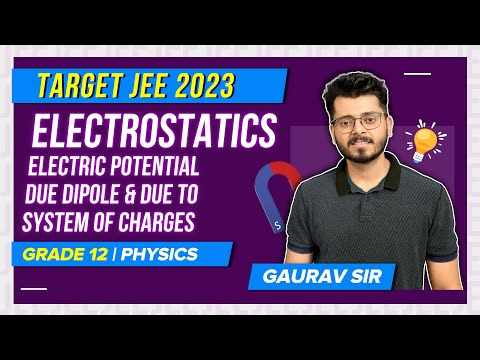s

#### Electrostatics Class 12 Physics JEE Mains Important Questions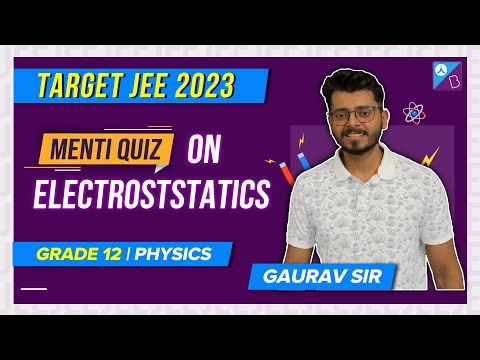#### Top 10 Important Questions of Electrostatic Potential and Capacitance Class 12 Physics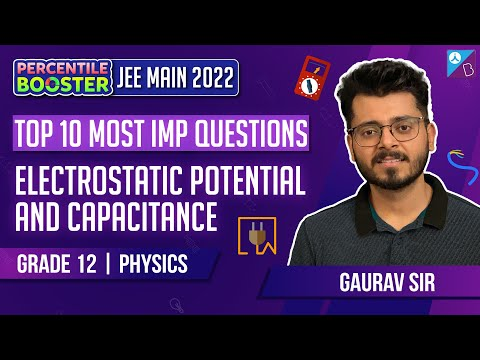s

#### Electric Field Class 12 Physics Practice Problemss

#### Electric Potential Class 12 Physics Practice Problemss

Stay tuned to BYJU’S and Fall in Love with Learning!